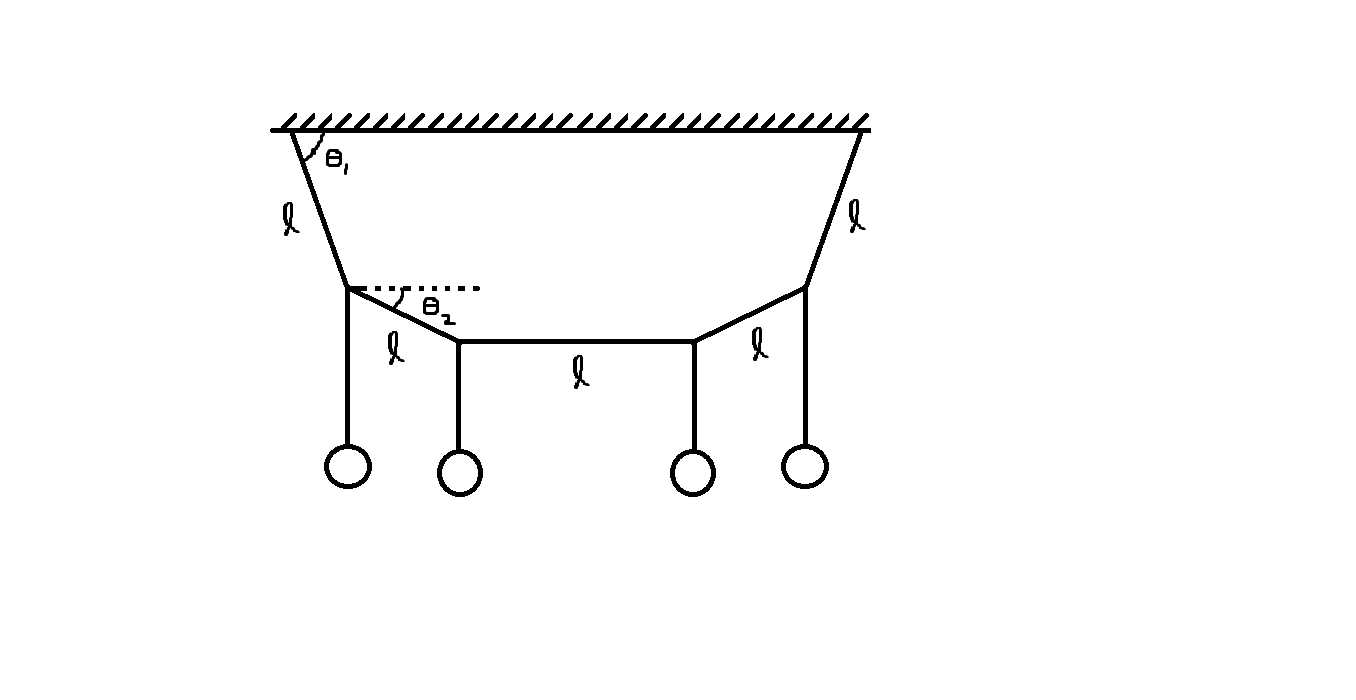# JEE Mains Mechanics (Equilibrium) 2Four identical metal balls are hanging from a light string of length $5l$ at equally placed points as shown in figure. The ends of the string are attached to a horizontal fixed support. The middle section of the string is horizontal. Calculate $\theta_2$ in degrees if $\theta_1=\tan^{-1}2$.

###### This problem is a part of My Picks for JEE Mains 1
×

Problem Loading...

Note Loading...

Set Loading...